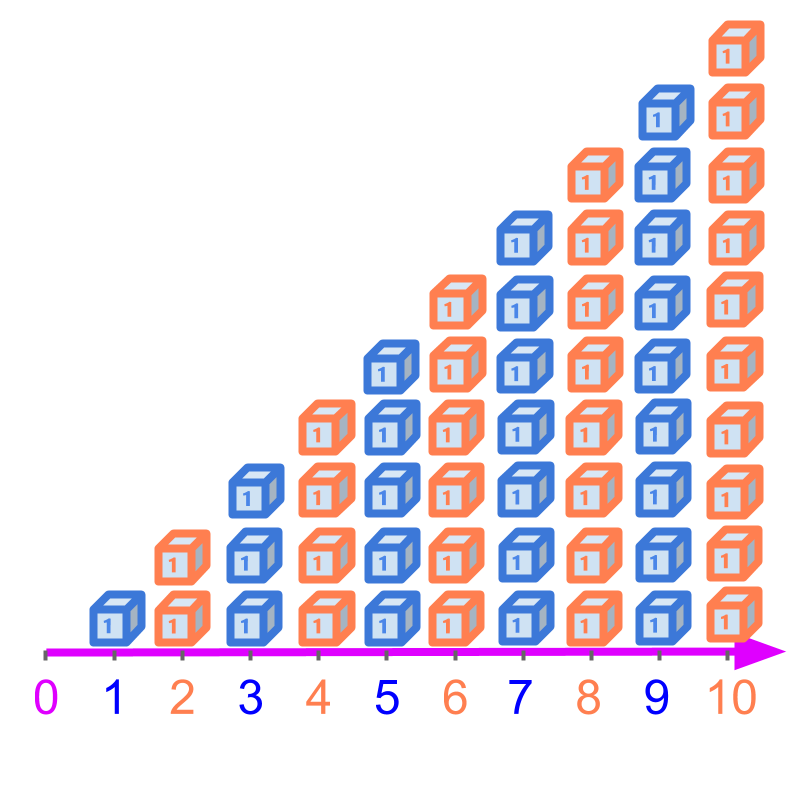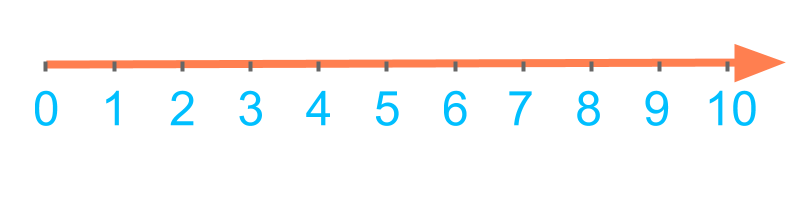maths > wholenumbers

Predecessor and Successor (Whole Numbers)

what you'll learn...

overview

The following are explained.

•  the number preceding in the order is the predecessor of a given number.

•  the number following in the order is the successor of a given number.

The predecessor and successor are explained with the number-line too.

before and afterWhole numbers are ordered in a specific sequence. This is represented by the number line.

Predecessor is the number prior to a number in the order.

Successor is the number that follows a number in the order.

The predecessor of 3$3$ is 2$2$.
The successor of 3$3$ is 4$4$.

The word "predecessor" means: something that comes before or prior in the order.

"pre" means "beforehand" and "decessor" is derived from a word meaning "that departed".The numbers are ordered 1,2,3,4,$1 , 2 , 3 , 4 , \cdots$.

Predecessor of a given number is the number that comes before or prior to the given number.

The predecessor of 3$3$ is $2$.
The predecessor of $4$ is $3$.

The word "successor" means: something that comes after or follows in the order.

The numbers are ordered $1 , 2 , 3 , 4 , \cdots$.

Successor to a given number is the number that follows the given number.

The successor of $3$ is $4$.
The successor of $4$ is $5$.

Predecessor: The number that comes before a given number is the predecessor of the given number. The predecessor is one less than the given number.

Successor: The number that comes after a given number is the successor of the given number. The successor is one greater than the given number.

examples

What is the successor of $9$?

The answer is '$10$'. The number-line continues after $9$ with $10 , 11 , 12 , \cdots$.

What is the predecessor of $13$?

The answer is '$12$'.

What is the predecessor of $233$?

The answer is '$232$'.

What is the successor of $233$?

The answer is '$234$'

What is the predecessor of $1$?

The answer is '$0$'.

summary

Predecessor: The number that comes before a given number is the predecessor of the given number. The predecessor is one less than the given number.

Successor: The number that comes after a given number is the successor of the given number. The successor is one greater than the given number.Examples:

•  predecessor of $3$ is $2$

•  sucessor of $3$ is $4$

Outline